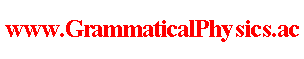since 2006 Help　Sitemap < Forum > < Definition > < Abstracts for the Meetings Held by the Physical Society > < JPS 2010 Spring Meeting > 20pBJ-1What status does an action functional have in the new grammar version of quantum theory? http://www.grammaticalphysics.ac/ / Yuuichi Uda---Let S denote an action functional.Then functional Φ defined as Φ[χ]＝exp{(i/h)S[χ]} is a function of the same type as a functional representing a quantum history of my new grammar version of quantum theory(JPS 2006 Spring Meeting 27pXA-6) in the meaning that it maps each function representing a classical history onto a complex number.Accordingly, it is relevant to ask what quantum history of the new grammar version of quantum theory is represented by Φ and to ask whether Φ is a solution of the new grammar version of the Schrödinger equation(JPS 2007 Spring Meeting 28pSL-11) or not.After actual calculation, I found that Φ is not a solution of the new grammar version of the Schrödinger equation.So, it may not be very important to investigate what quantum history is represented by Φ although Φ must represent some quantum history.Then what significance does Φ or S have in the new grammar version of quantum theory?This presentation is to propose this problem.It is not a presentation of any solution for this problem.By making an equation which Φ is a solution of instead of the new grammar version of Schrödinger equation, are we led to some new grammar other than the new grammar proposed by me?I found that Φ defined above is a solution of the equation∫dt[ihδ／δχ(t)－dV(χ(t))／dχ(t)]Φ[χ]＝0,when we use the circular time(JPS 2009 Autumn Meeting 13pSH-3).Here I assumed that S[χ]＝∫dt{(m/2)[dχ(t)／dt]2－V(χ(t))}.I do not know the physical meaning of this equation.I am interested in the point that rewriting an equation to the form of variation principle can be thought of as a deed transforming the equation to a function(functional).For example,rewriting the equation of motion of classical mechanics to the form of variation principle is the deed transforming the equation of motion to an action functional.If being zero of variation has only to be equivalent to the equation of motion, there are innumerable such functionals other than the action functional.As far as classical mechanics is concerned, the arbitrary part of such a functional has no physical meaning.However, path integral method suggests that the whole of an action functional has physical meaning in quantum mechanics.So, we should study the validity of the ladder:an equation → a function(functional) → another equation,as a method to take the grammar of our theory a stage farther. --- This article is a rewrite of the following article. JPS 2010 Spring Meeting@Abstracts for the Meetings Held by the Physical Society@Definition of Grammatical Physics@Grammatical Physics@Forum@Vintage(2008-2014) Author Yuichi Uda, Write start at 2015/05/25/19:26JST, Last edit at 2015/05/25/19:26JST